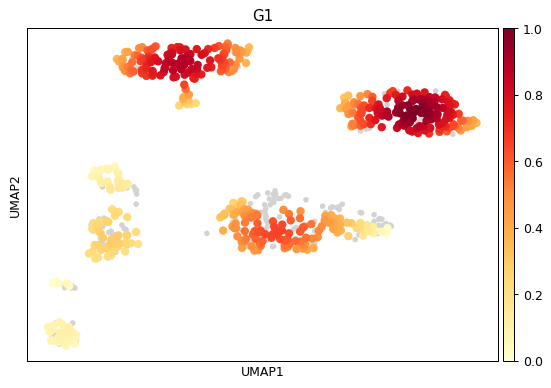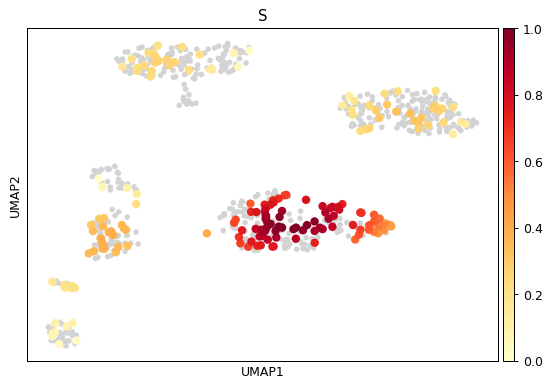# scanpy.tl.embedding_density¶

Calculate the density of cells in an embedding (per condition).

Gaussian kernel density estimation is used to calculate the density of cells in an embedded space. This can be performed per category over a categorical cell annotation. The cell density can be plotted using the `sc.pl.embedding_density()` function.

Note that density values are scaled to be between 0 and 1. Thus, the density value at each cell is only comparable to other densities in the same condition category.

This function was written by Sophie Tritschler and implemented into Scanpy by Malte Luecken.

Parameters
adata : `AnnData``AnnData`

The annotated data matrix.

basis : `str``str` (default: `'umap'`)

The embedding over which the density will be calculated. This embedded representation should be found in `adata.obsm['X_[basis]']``.

groupby : (default: `None`)

Keys for categorical observation/cell annotation for which densities are calculated per category. Columns with up to ten categories are accepted.

key_added : (default: `None`)

Name of the `.obs` covariate that will be added with the density estimates.

components : (default: `None`)

The embedding dimensions over which the density should be calculated. This is limited to two components.

Return type
Returns

Updates `adata.obs` with an additional field specified by the `key_added` parameter. This parameter defaults to `[basis]_density_[groupby]`, where where `[basis]` is one of `umap`, `diffmap`, `pca`, `tsne`, or `draw_graph_fa` and `[groupby]` denotes the parameter input. Updates `adata.uns` with an additional field `[key_added]_params`.

Examples

```import scanpy as sc```sc.pl.embedding_density(